Click to Chat

1800-1023-196

+91-120-4616500

CART 0

• 0

MY CART (5)

Use Coupon: CART20 and get 20% off on all online Study Material

ITEM
DETAILS
MRP
DISCOUNT
FINAL PRICE
Total Price: Rs.

There are no items in this cart.
Continue Shopping• Complete JEE Main/Advanced Course and Test Series
• OFFERED PRICE: Rs. 15,900
• View Details

Revision Notes on Electrostatics

Electrostatics:- It is a branch of physics that deals with the phenomena and properties of stationary or slow-moving electric charges with no acceleration.

Coulomb’s Law:- It states that the electro-static force of attraction or repulsion between two charged bodies is directly proportional to the product of their charges and varies inversely as the square of the distance between the two bodies.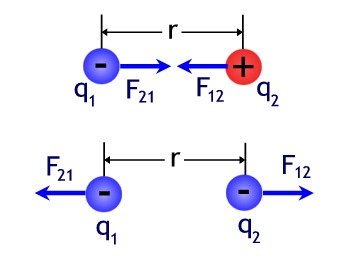F = Kq1q2/r2

Here, K = 1/4πε0 = 9×109 Nm2C-2 (in free space)

Relative Permittivity (εr):-The relative permittivity (εr) of a medium is defined as the ratio between its permittivity of the medium (ε) and the permittivity (ε0) of the free space.

εr = ε/ε0

Coulomb force in vector form:- The force on charge q1 due to q2 is,

If q1q2>0, R.H.S is positive.

If q1q2<0, a negative sign from q1q2 will change  and .  The relation will again be true, since, in that case  have same directions.

Unit of Charge:-

C.G.S, q = ±1 stat-coulomb

S.I, q = ±1 Coulomb

Relation between coulomb and stat-coulomb:-

1 coulomb = 3×109 stat-coulomb

1 coulomb =(1/10) ab-coulomb (e.m.u of charge)

Dielectric constant:- The dielectric constant (εr) of a medium can be defined as the ratio of the force between two charges separated by some distance apart in free space to the force between the same two charges separated by the same distance apart in that medium.

So, εr = ε/ε0 = F1/F2

Here, F1 and F2 are the magnitudes of the force between them in free space and in a medium respectively.

Charges:-

Line charge, λ = q/L

Surface charge, σ = q/A

Volume charge, ρ = q/V

Electric field () :- The strength of an electric field is measured by the force experienced by a unit positive charge placed at that point. The direction of field is given by the direction of motion of a unit positive charge if it were free to move.

Unit of Electric field:- E = [Newton/Coulomb] or [Joule/(Coulomb) (meter)]

Electric lines of force:- An electric line of force is defined as the path, straight or curved, along which a unit positive charge is urged to move when free to do so in an electric field. The direction of motion of unit positive charge gives the direction of line of force.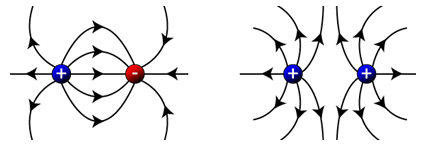Properties:-

(a) The lines of force are directed away from a positively charged conductor and are directed towards a negatively charged conductor.

(b) A line of force starts from a positive charge and ends on a negative charge. This signifies line of force starts from higher potential and ends on lower potential.

Electric field intensity due to a point charge:- E = (1/4πε0) (q/r2)

Electric field Intensity due to a linear distribution of charge:-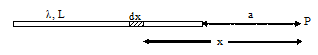?(a) At point on its axis.

E = (λ/4πε0) [1/a – 1/a+L]

Here, λ is the linear charge density.

(b) At a point on the line perpendicular to one end.

?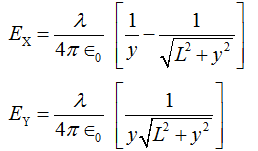Here λ is the line charge.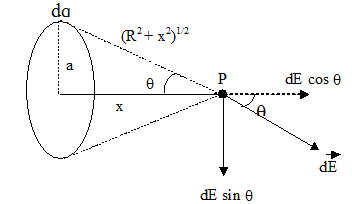Electric field due to ring of uniform charge distribution:-

At a point on its axis, E = (1/4πε0) [qx/(a2+x2)3/2]

Electric field due to uniformly charged disc:-Here σ is the surface charge.

Electric field due to thin spherical shell:-(a) Eout = (1/4πε0) (q/r2)

(b) Ein = 0

Electric field of a non-conducting solid sphere having uniform volume distribution of charge:-

?(a) Outside Point:- Eout = (1/4πε0) (Q/r2)(b) Inside Point:- Ein = (1/4πε0) (Qr/R3)

(c) On the Surface:- Esurface = (1/4πε0) (Q/R2)

Here, Q is the total charge

Electric field of a cylindrical conductor of infinite length having line charge λ:-(a) Outside the cylinder:- E = λ/2πε0r

(b) Inside the cylinder:- E = 0

Electric field of a non-conducting cylinder having uniform volume density of charge:-

(a) Outside the cylinder:- E = λ/2πε0r

(b) Inside a point:- E = ρr/2ε0

Electric field of an infinite plane sheet of charge surface charge (σ) :- E = σ/2ε0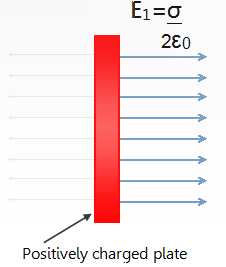Electric field due to two oppositely infinite charged sheets:-

(a) Electric field at points outside the charged sheets:-

EP = ER = 0

(b) Electric field at point in between the charged sheets:-

EQ = σ/ε0

Electric Dipole:- An electric dipole consists of two equal and opposite charges situated very close to each other.

Dipole Moment:- Dipole moment () of an electric dipole is defined as the product of the magnitude of one of the charges and the vector distance from negative to positive charge.

Unit of Dipole Moment:- coulomb meter (S.I), stat coulomb cm (non S.I)

Electric field due to an electric dipole:-

?(a) At any point on the axial line:-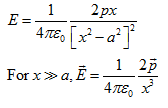(b) At a point on the equatorial line (perpendicular bisector):-(c) At any point:-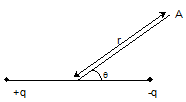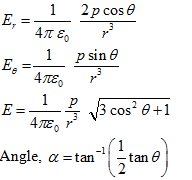Torque () acting on a electric dipole  in a uniform electric field (E):-

= pE sinθ

Here, p is the dipole moment and θ is the angle between direction of dipole moment and electric field E.

Electric Flux:- Electric flux ?E for a surface placed in an electric field is the sum of dot product of   and  for all the elementary areas constituting the surface.

Gauss Theorem:- It states that, for any distribution of charges, the total electric flux linked with a closed surface is 1/ε0 times the total charge with in the surface.Electric field (E) of an infinite rod at a distance (r) from the line having linear charge density (λ):-

E = λ/2πε0r

The direction of electric field E is radially outward for a line of positive charge.

Electric field of a spherically symmetric distribution of charge of Radius R:-

(a) Point at outside (r > R):- E = (1/4πε0) (q/r2), Here q is the total charge.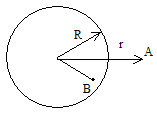(b) Point at inside (r < R):- E = (1/4πε0) (qr/R3), Here q is the total charge.

Electric field due to an infinite non-conducting flat sheet having charge σ:-

???E = σ/2ε0

This signifies, the electric field near a charged sheet is independent of the distance of the point from the sheet and depends only upon its charge density and is directed normally to the sheet.

Electric field due to an infinite flat conductor carrying charge:-

?E= σ/ε0

Electric pressure (Pelec) on a charged conductor:-

Pelec = (½ε0) σ2### Course Features

• 728 Video Lectures
• Revision Notes
• Previous Year Papers
• Mind Map
• Study Planner
• NCERT Solutions
• Discussion Forum
• Test paper with Video Solution